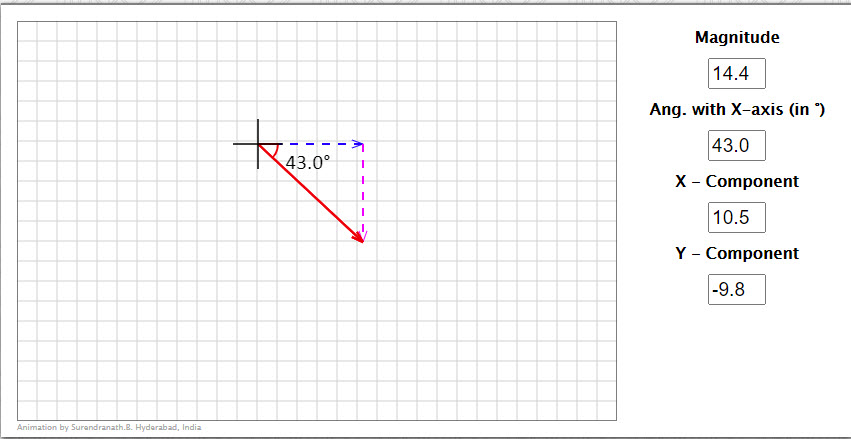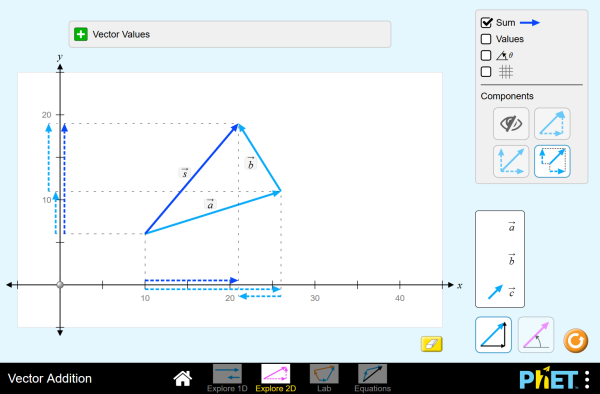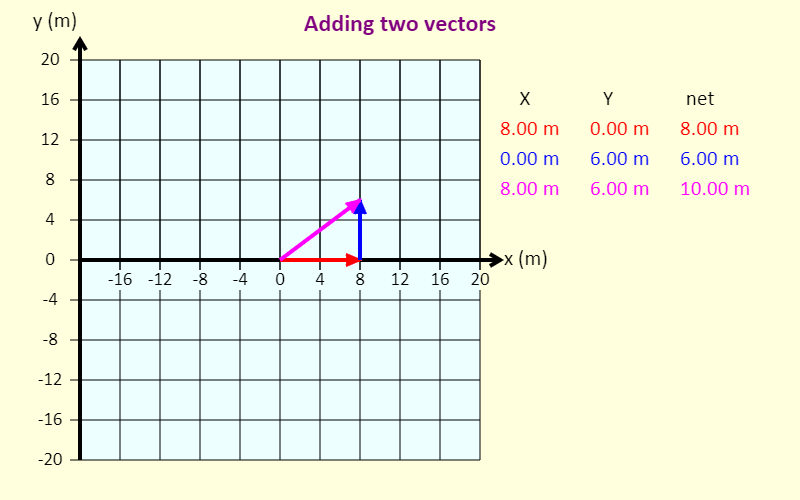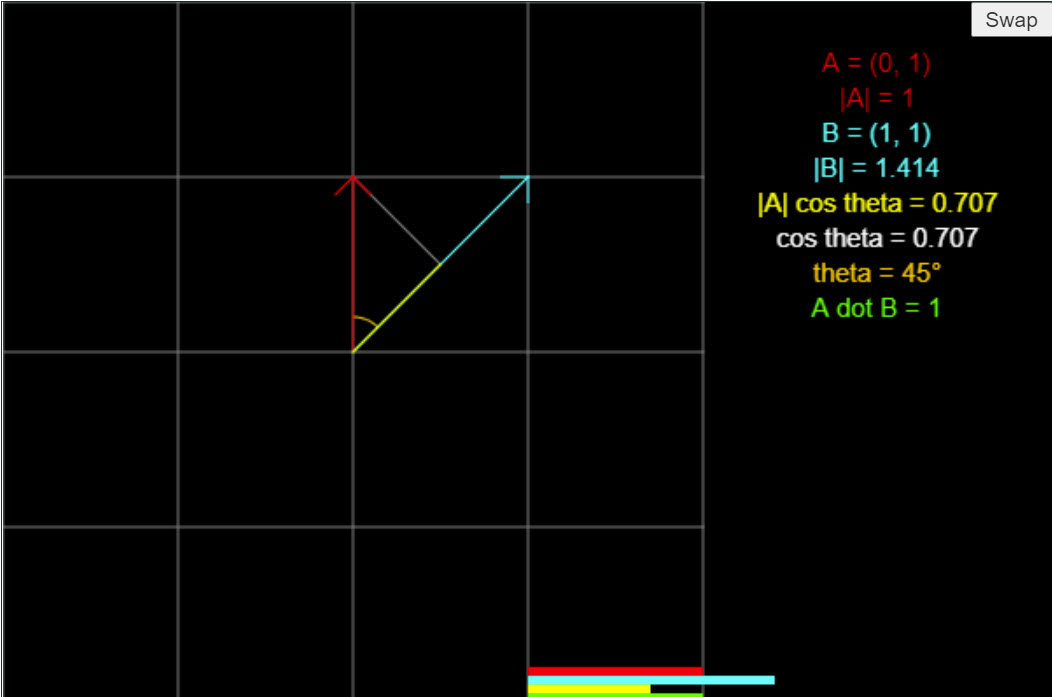# Vectors

Student Learning Objectives
Lessons / Lecture Notes
Important Equations
Example Problems
Applets and Animations

Student Learning Objectives

• To understand the basic properties of vectors.
• To add and subtract vectors both graphically and using components.
• To be able to decompose a vector into its components and to reassemble vector components into a magnitude and a direction.
• To recognize and use the basic unit vectors.
• To work with tilted coordinate systems.

Lessons / Lecture Notes

The Physics Classroom (conceptual)

PY105 Notes from Boston University (algebra-based):

Physics 2A notes from Dr. Bobby W.S. Lau (algebra-based)

HyperPhysics (calculus-based)

Physics 4A notes from Dr. Bobby W.S. Lau (calculus-based)

Important Equations
(for algebra-based Physics)

Example Problems

Example Problems for algebra-based physics (from College Physics 2nd Edition by Knight, Jones, and Field)

Example Problems (Vectors & Motion in Two Dimension)
Solutions to Example Problems (Vectors & Motion in Two Dimensions)

Example Problems for calculus-based physics (from Physics for Scientists and Engineers 4th Edition by Knight):

Example Problems (Vectors and Coordinate Systems)
Solutions to Example Problems (Vectors and Coordinate Systems)

Applets and Animations

 Vector ComponentsVector can be defined or drawn. To define make entries in the text fields. You can enter values for magnitude and direction or component values. After entering the values in the text fields, press enter to draw the new vector. To draw, click/tap anywhere and drag. Vector AdditionExplore vectors in 1D or 2D, and discover how vectors add together. Specify vectors in Cartesian or polar coordinates, and see the magnitude, angle, and components of each vector. Experiment with vector equations and compare vector sums and differences. Resultant of Forces (Addition of Vectors)This app deals with forces exerted on a body (assumed as point-sized). You can vary the number of single forces by using the choice box at the ride side. It is possible to change the sizes and directions of these forces (blue arrows) by dragging the arrowheads to the intended positions with pressed mouse button. If you want to know the total force which is exerted on the body, you have to carry out a vector addition. As soon as you have clicked on the button "Find out resultant" the program will show you the necessary parallel translations of the force arrows and then draw the arrow of the resultant (red). The construction can be cleared by a mouse click on the lower button. Vector AdditionWith this nice applet, you can visually add 2, 3, 4, or 5 vectors together. Vector Addition (Adding Two Vectors)The simulation shows two vectors and their sum, which we call the resultant vector. The x and y components of each of the vectors are also shown. Dot ProductThis applet demonstrates the dot product, which is an important concept in linear algebra and physics. The goal of this applet is to help you visualize what the dot product geometrically.